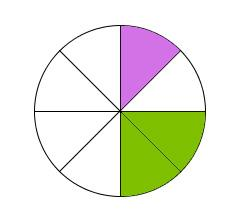# Denominator 2858

Which same integer must we add to the reader and the denominator of the fraction 37/73 so that the fraction equals one-half?

x =  -1

### Step-by-step explanation:Did you find an error or inaccuracy? Feel free to write us. Thank you!

Tips for related online calculators
Need help calculating sum, simplifying, or multiplying fractions? Try our fraction calculator.
Do you have a linear equation or system of equations and looking for its solution? Or do you have a quadratic equation?